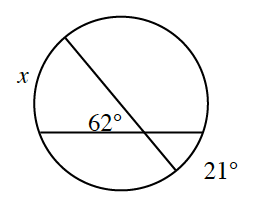### Home > CCG > Chapter 11 > Lesson 11.2.3 > Problem11-120

11-120.Copy the diagram at right onto your paper. Use the process from problem 11‑111 to find the measure of $x$. Show all work.

In problem 11-111, it was established that the vertical angles are equal to the average of the two arcs.

Therefore $\frac{x+21°}{2}=62°$